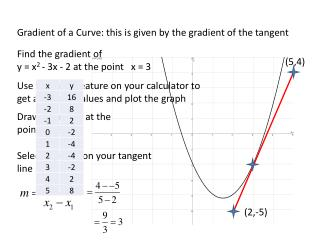DownloadDownload PresentationGradient of a Curve: this is given by the gradient of the tangent

# Gradient of a Curve: this is given by the gradient of the tangent

Download Presentation## Gradient of a Curve: this is given by the gradient of the tangent

- - - - - - - - - - - - - - - - - - - - - - - - - - - E N D - - - - - - - - - - - - - - - - - - - - - - - - - - -
##### Presentation Transcript

1. Gradient of a Curve: this is given by the gradient of the tangent Find the gradient of y = x2 - 3x - 2 at the point x = 3 (5,4) Use the table feature on your calculator to get a table of values and plot the graph Draw a tangent at the point x = 3 Select 2 points on your tangent line (2,-5)

2. Gradient of a Curve: this is given by the gradient of the tangent Find the gradient of y = x2 - 3x - 2 at the point x = -1 (-1.5,4) Draw a tangent at the point x = -1 Select 2 points on your tangent line (0,-3.5)

3. Finding the Equation of the tangent Find the equation of the tangent to y = x2 - 3x - 2 at the point x = 3 Using y = mx + C From slide 1 m = 3 and x = 3 To find y use the equation y = x2-3x-2 with x = 3 y = 32-33-2 = -2 So y = -2

4. Finding the Equation of the tangent Find the equation of the tangent to y = x2 - 3x - 2 at the point x = 3 Using y = mx + C m = 3 and x = 3 , y = -2 y = 3x-11 Using y = mx + C -2 = 33 + C -2 = 9 + C Forwards C + 9 = -2 Backwards -2-9 = C C = -11 So the equation of the tangent is y = 3x-11

5. Checking on the calculator Enter y1 = x2-3x-2 and y2 = 3x-11 Now do the questions on pg 30 in your notes